University of Virginia, Department of Computer Science CS551: Security and Privacy on the Internet, Fall 2000

 Problem Set 1: Classical Ciphers Selected Answers

Average: 88.4/100
Problem 1: 9.8/10, Problem 2: 15.7/20, Problem 3: 29.3/35, Problem 4: 33.6/35 (+ 20)

### 1. Security Principles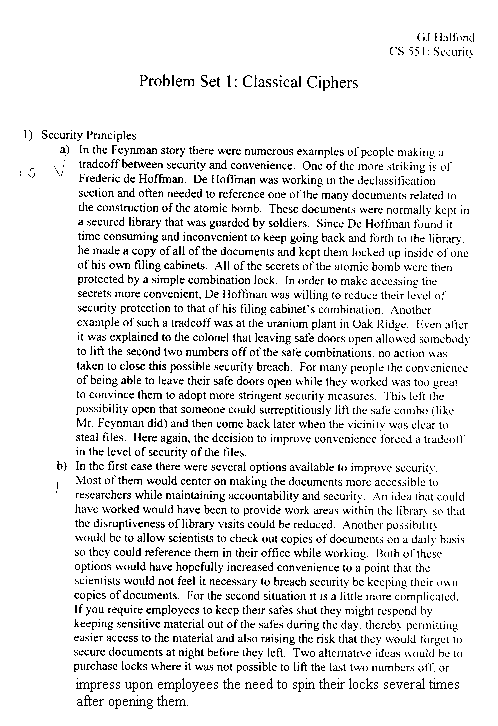### 2. Cryptogram

(20) Decrypt the encrypted message below. It is known to be English. Show your work and explain the process you used, including any programs you wrote.
```VPTGN YIFWF HDETO UNTJS NOHKK VDGUZ IOCCH NVIPZ UYTQZ
UQECK OKETK OKETK UAECF XCAFH YJPNW AJTQS FGTJS NORQM
VGEVG BJIUL NCEVZ CIGWH CITQZ CNOHX CXECF XCEFA XITGN
YIBQL BZRVG MZTVZ YXOOT CIAVA II
```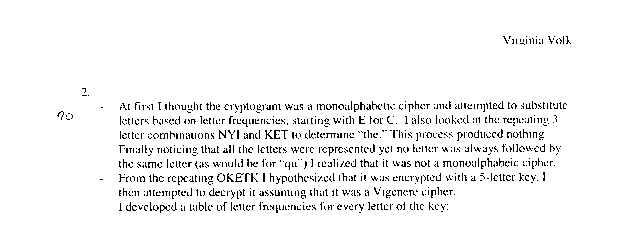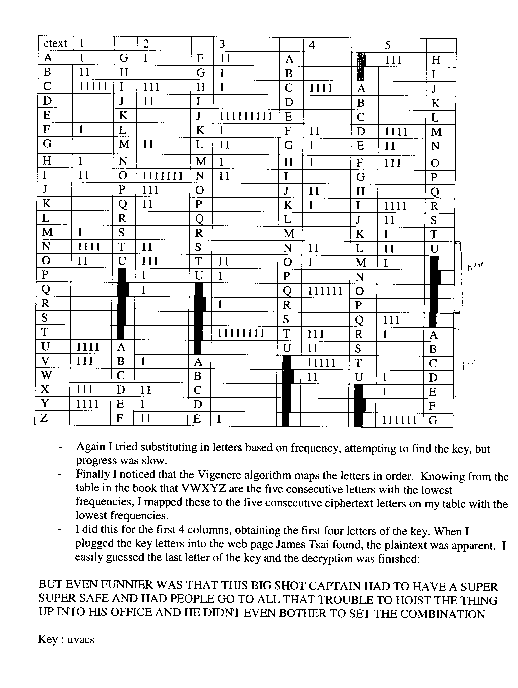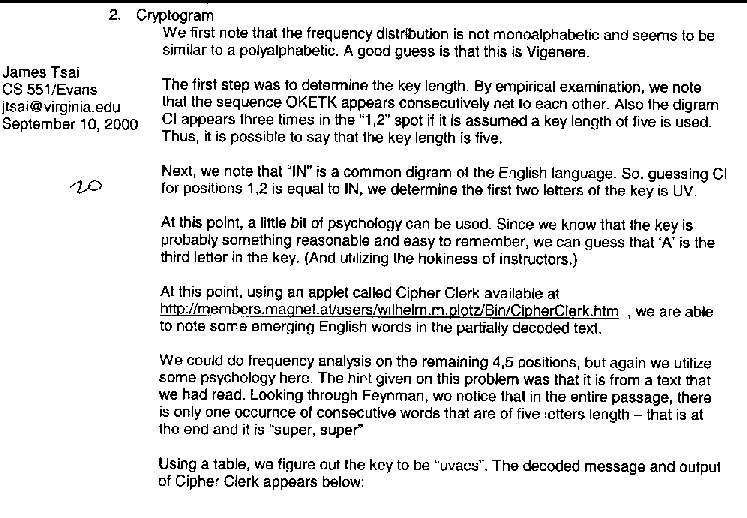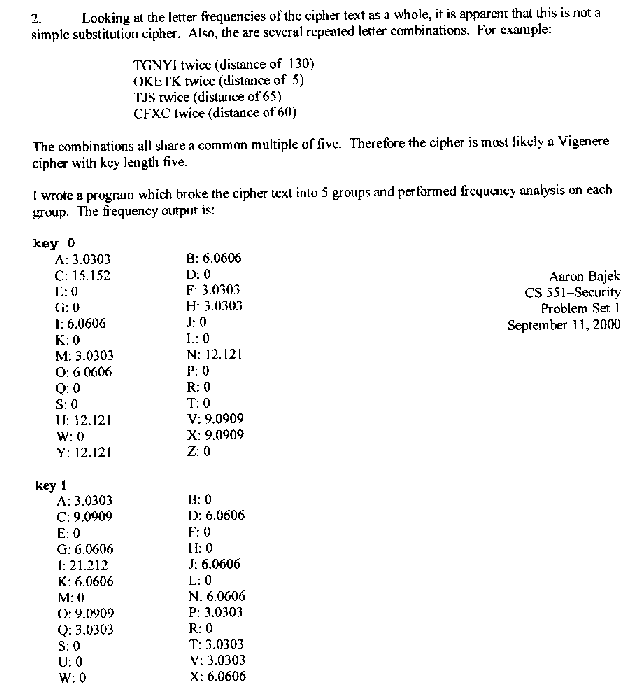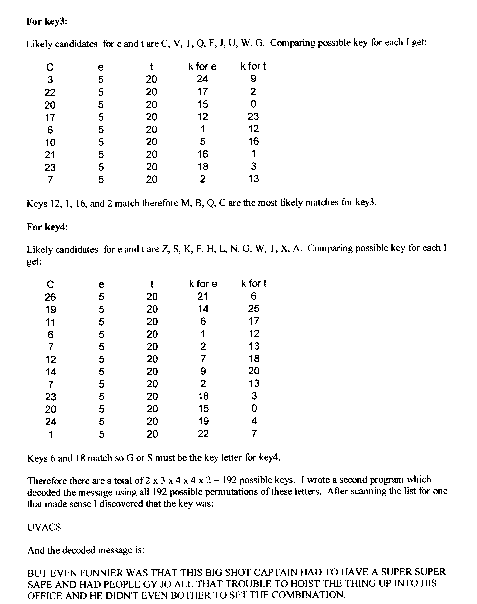### 3. Jefferson's Cipher

a. (5) Assuming the arrangement of the letters on the wheels is known by a potential attacker (that is, the only secret is the order of the wheels on the spindle), how large is the search space for a brute-force attack?

Answer: 26! (any wheel could be first, any other wheel could be second, ...)

b. (5) If the arrangement of letters on the wheels is also unknown to the attacker, how large is the search space for a brute-force attack?

Answer: (25!)^36 (approximately 10^56). Each wheel can be arranged 25! different ways. Since the wheel rotates, it doesn't matter which letter is choosen first. Each wheel is independent, so we raise it to the 36th power.

c. (5) Suggest a known-plaintext attack: determine the key given known wheels and a known plaintext. Estimate how much plaintext is necessary for a successful attack.

d. (10) Describe a ciphertext-only attack. You may assume the wheels are known.

Many different interesting solutions were given, although no one has successfully demonstrated their solution in practics. No solutions will be provided for c and d until the challenge problem is solved.

e. (10) Jefferson Wheel operators tend to be careless and always choose the 3rd line above the plaintext for the ciphertext. How much easier is a ciphertext-only attack if this is done?

Answer: If the 3rd line is always choosen, we can slice the ciphertext to every 36th letter, and use simple frequency analysis to derive the key or plaintext.

Maury Bond, Secret Agent 000, wants to give the directions to the super ray gun to his colleagues Sly McCraken, Cript O'Hacker and Trey Tor. The message M is n-bits long. He suspects one of them may be a double agent, so he divides the message as follows:
• Sly gets K_1 an n-bit random sequence.
• Cript gets K_2 an n-bit random sequence.
• Trey gets C = K_1 XOR K_2 XOR M.
a. (5) How can Sly, Cript and Trey determine M?

Answer: M = K_1 XOR K_2 XOR (K_1 XOR K_2 XOR M). Sly, Cript and Trey XOR all their keys to obtain the plaintext.

b. (10) Is the scheme secure? Argue convincingly that either (1) it is secure - no two people can determine any bit of M with probability greater than 1/2; or (2) is it insecure - two peoople can conspire to determine a bit of M with probability greater than 1/2.

Answer: Yes. The scheme is secure if we can show no two people can obtain the probability of any bit with p > 1/2.

K_1 and K_2 are random bit strings, so Sly or Cript alone have no information about M.

Trey has T = K_1 XOR K_2 XOR M. The probability that T[i] is 0 is:

```p (T[i] = 0) = p (K_1[i] = 0) * p(K_2[i] = 0) * p(M[i] = 0)
+ p (K_1[i] = 1) * p(K_2[i] = 1) * p(M[i] = 0)
+ p (K_1[i] = 0) * p(K_2[i] = 1) * p(M[i] = 1)
+ p (K_1[i] = 1) * p(K_2[i] = 0) * p(M[i] = 1)

all the key bit probabilities are 1/2:

= .5 * .5 * p(M[i] = 0) + .5 * .5 * p(M[i] = 0)
+ .5 * .5 * p(M[i] = 1) + .5 * .5 * p(M[i] = 1)
= .5 * p(M[i] = 0) + .5 * p(M[i] = 1)
= .5 * (p(M[i] = 0) + p(M[i] = 1)) = .5
```
In conspiracy with Sly, Trey can calculate ST = K_2 XOR M. We can show p (ST[i] = 0) = .5 using a similar proof to what we did in class for the one-time pad perfect cipher. Similarly for Cript and Trey conspiring to produce K_1 XOR M.

c. (10) Sly, Cript and Trey gather in Borneo to combine their messages and track down the super ray gun. Sly reveals K_1, Cript reveals K_2, and Trey reveals a n-bit random sequence. They combine the keys to determine M, but a meaningless bit sequence results. Sly and Cript leave the island befuddled, while Trey uses K_1, K_2 and C (which he kept to himself) to construct M and locate the super ray gun for himself. What could be done to prevent this?

Answer: This question was intended to get you thinking about the differences between the math of cryptology and the reality of security. Even a provably perfect scheme can break if it is used carelessly in practice!

One approach is to not reveal keys to the other agents. Sly, Cript and Trey each secretly type their keys into a black box. After this, the black box reveals the XOR of the three inputs. Something must be done (e.g., carry guns) to ensure the last agent doesn't run off with the black box instead of showing the result to the other agents.

A similar idea is to reveal key bits one at a time in random order. A cheater can only get a few bits before the others notice the results are gibberish.

Other approaches catch cheaters. We could give each person a few randomly selected bits of the other keys. This breaks the prefect cipher property, since two agents could now conspire to determine those key bits. But, if only 1% of the bits were selected, this would be unlikely to provide enough information to locate the super ray gun. A cheater would be caught if they reveal a bit that doesn't match the shared key bits.

Later in the class, we will see some more satisfying ways to do this using one-way hashing functions.

d. (10) Maury is worried that if one agent is killed, the others will never be able to find the super ray gun. He believes it is unlikely that more than one agent will be killed, however. Suggest a scheme Maury can use to distribute the message so that any two agents can combine their shares to determine the message, but no one agent alone can decipher the message.

Answer: The simplest scheme is to give each agent two keys:

```Sly:     K_1 and K_2
Cript:   K_2 and (K_1 XOR K_2 XOR M)
Trey:    K_1 and (K_1 XOR K_2 XOR M)
```
Any single agent has no information (we can redo the proof from part b), but any two agents can combine their keys to get the message.

e. (up to 20 bonus points) Suggest a scheme that scales to 99 out of 100 agents that doesn't require an unreasonable about of key data.

Answer: This question was a bit vague since I didn't specify what an "unreasonable amount of key data" was. The obvious extension of the previous answer would require 100! keys (which is clearly unreasonable).

Jae Pak suggests:

```Agent_001 gets K_001 and K_001 XOR K_002 XOR ... K_099 XOR K_100 XOR M XOR K_100
Agent_002 gets K_002 and K_001 XOR K_002 XOR ... K_099 XOR K_100 XOR M XOR K_001
...
Agent_100 gets K_100 and K_001 XOR K_002 XOR ... K_099 XOR K_100 XOR M XOR K_099
```
So, each agent has enough information to reconstruct M with every other key except one. Agent 007 can reconstruct the message with every agent's key except Agent 006's.

This is a good scheme, but the desired security properties are not quite maintained. Any 2 adjacent agents can recover a third agent's key: agent 001 and agent 002 can conspire to determine K_100:

```    K_100 = (Agent_002's piece XOR Agent_001's piece XOR K_001)
```
Similarly, agent 002 and agent 003 can recover K_001. So, a conspiracy of 67 agents can determine the message!

I don't believe there is any provably perfectly secure way to do this --- that is, any x-2 agents can produce no information, x-1 agents can produce M, and the total number of key bits is O (x * n) where n is the size of M. There's 50 bonus points for anyone who either produces such a scheme or proves that no such scheme exists.

There are many schemes for sharing secrets that do not use XOR and are not provably perfect. One approach is to use a system of linear equations. See Bruce Schneier, Applied Cryptography: Protocols, Algorithms, and Source Code in C, 2nd Edition, Section 23.2 for details.